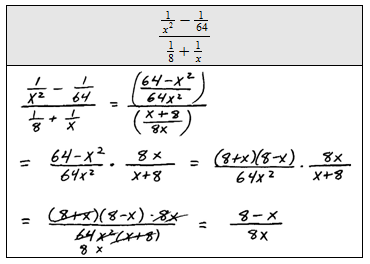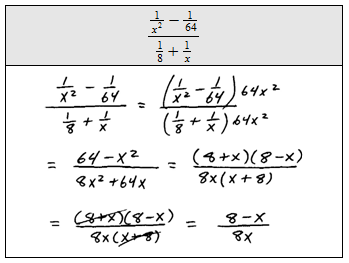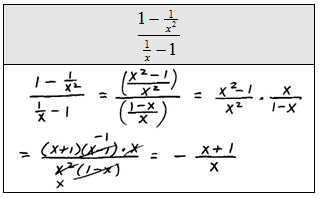## Algebra

Showing posts with label divide. Show all posts
Showing posts with label divide. Show all posts

### Multiplying and Dividing Radical Expressions

As long as the indices are the same, we can multiply the radicands together using the following property.Since multiplication is commutative, you can multiply the coefficients and the radicands together and then simplify.

Multiply.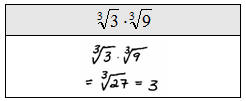Take care to be sure that the indices are the same before multiplying.  We will assume that all variables are positive.

Simplify.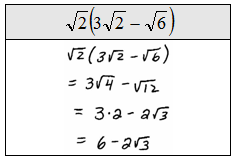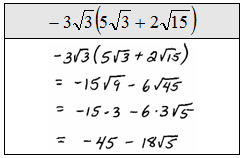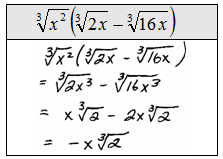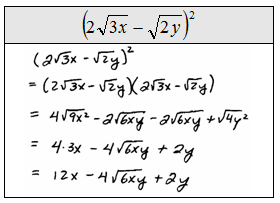Divide radicals using the following property.Divide. (Assume all variables are positive.)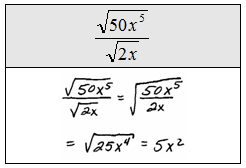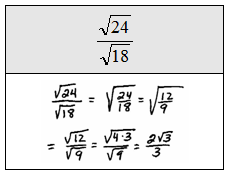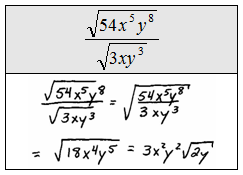Rationalizing the Denominator
A simplified radical expression cannot have a radical in the denominator.  When the denominator has a radical in it, we must multiply the entire expression by some form of 1 to eliminate it.  The basic steps follow.

Rationalize the denominator: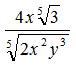Multiply numerator and denominator by the 5th root of of factors that will result in 5th powers of each factor in the radicand of the denominator.Notice that all the factors in the radicand of the denominator have powers that match the index.  This was the desired result, now simplify.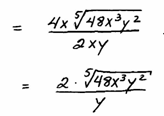Rationalize the denominator.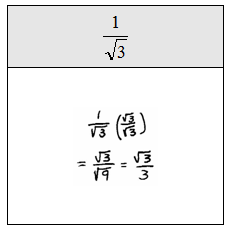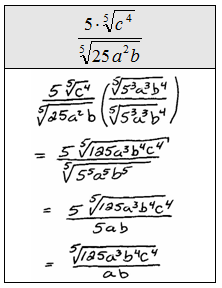This technique does not work when dividing by a binomial containing a radical.  A new technique is introduced to deal with this situation.

Rationalize the denominator:Multiply numerator and denominator by the conjugate of the denominator.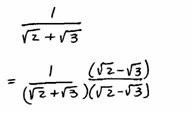And then simplify. The goal is to eliminate all radicals from the denominator.Rationalize the denominator.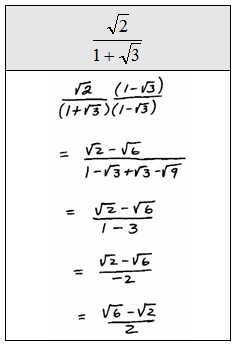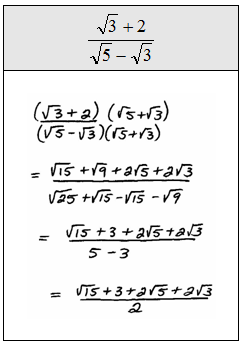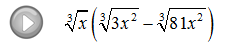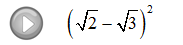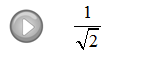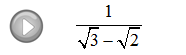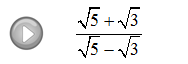### Complex Rational Expressions

It turns out that we have all the tools necessary to simplify complex algebraic fractions.

Rational Expressions and Equations Playlist on YouTube

The numerator and denominator of these rational expressions contain fractions and look very intimidating.  We will outline two methods for simplifying them.

Method 1: Obtain a common denominator for the numerator and denominator, multiply by the reciprocal of the denominator, then factor and cancel if possible.Method 2: Multiply the numerator and denominator of the complex fraction by the LCD of all the simple fractions then factor and cancel if possible.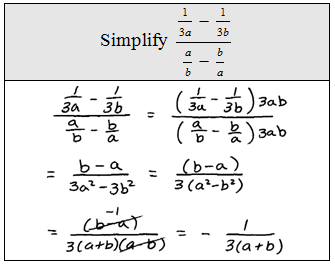To illustrate what happened after we multiplied by the LCD we could add an extra step.For the following solved problems, both methods are used. Choose whichever method feels most comfortable for you.

Simplify using method 1. Simplify using method 2.